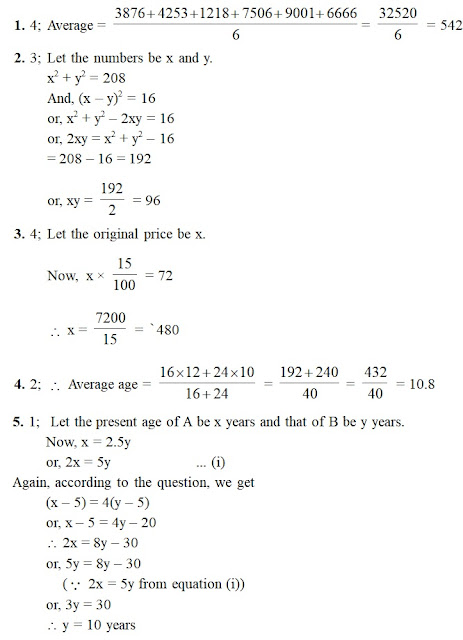Important Arithmetic Questions for RBI Astt.

1. What is the average of the following number series?
3876       4253         1218           7506         9001      6666
1) 5180                  2) 5260                  3) 5340
4) 5420                  5) 5500
1. The sum of the squares of two numbers is 208 and the square of their difference is 16. What is the product of the two numbers?
1) 72                       2) 84                       3) 96
4) 108                    5) None of these
1. If the price of an item is increased by 15% it increases by `72. What is the original price of the item?
1) `420                   2) `440                   3) `460
4) `480                   5) None of these
1. A class has 16 boys and 24 girls. The average age of the boys is 12 years and that of the girls is 10 years. What is the average age of the whole class? (in years)
1) 10.6                   2) 10.8                   3) 11
4) 11.2                   5) 11.4
1. The age of A five years ago was four times the age of B. At present, A’s age is 2.5 times the age of B. What is the present age of B?
1) 10 years            2) 15 years            3) 20 years
4) 25 years            5) None of these
1. What value should come in place of question mark (?) in the following number series?
2                7                28              45              86         ?
1) 131                    2) 127                    3) 121
4) 115                    5) None of these
1. Mr X sells an article for `2668 and earns a profit of 15%. What is the cost price of the article?
1) `25800              2) `24400              3) `23200
4) `22500              5) `22000
1. 44% of a number is less than its 58% by 98. What is 61% of that number?
1) 427                    2) 488                    3) 366
4) 549                    5) None of these
1. What is the least number to be subtracted from 5783 to make it a perfect square?
1) 5                         2) 7                         3) 9
4) 11                       5) 3
1. 25 persons can fill 40 boxes in 6 days. How many persons are required to fill 48 boxes in 5 days?
1) 30                       2) 32                       3) 36
4) 42                       5) 48Share To: